## Helmholtz Differential Equation--Parabolic Coordinates

The Scale Factors are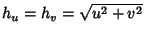,and the separation functions are,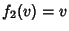,, given a Stäckel Determinant of. The Laplacian is(1)

Attempt Separation of Variables by writing(2)

then the Helmholtz Differential Equation becomes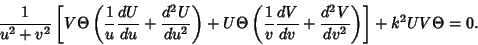(3)

Now divide by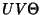,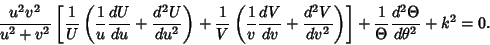(4)

Separating thepart,(5)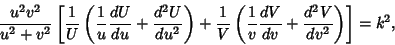(6)

so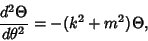(7)

which has solution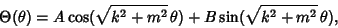(8)

and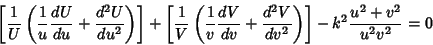(9)(10)

This can be separated(11)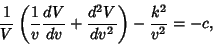(12)

so(13)(14)

References

Arfken, G. Parabolic Coordinates.'' §2.12 in Mathematical Methods for Physicists, 2nd ed. Orlando, FL: Academic Press, pp. 109-111, 1970.

Morse, P. M. and Feshbach, H. Methods of Theoretical Physics, Part I. New York: McGraw-Hill, pp. 514-515 and 660, 1953.